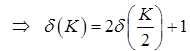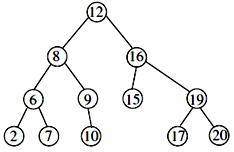# GATE Solved Paper 2017-19 - GATE 2017 Shift 2

>>>>>>>>GATE 2017 Shift 2

• A

θ(log logn)• B

θ(logn)• C

θ(√n)• D

θ(n)• Option : B
• Explanation :
T(n) = 2T(√n) + 1
Put n = 2K
T(2K) = 2T(2K/2) + 1
T(2K) = δ(K)By master's theorem
δ(K) = θ(K)
T(2K) = θ(K)
T(n) = θ(log n)   ∵ 2K = n

• A

54• B

45• C

55• D

58• Option : A
• Explanation :
Using Linearity of Expectation, we can write,
E[(X+2)2] = E[X2] + E[4X] + E
The Poisson distribution, mean and variance are same. Here Mean is given as 5. So variance should also be 5.
Also,
Variance = E[X2] – (E[X])2
5 = E[X2] – 25.
E[X2] = 30
Thus E[(X+2)2] = 30 + 4*5 + 4 = 54.

• A

θ(n√n)• B

θ(n2)• C

θ(n log n)• D

θ(n2 log n)• Option : C
• Explanation :
for i = 1
j will run from 1 to n by incrementing by '1' in each step 'j 'will run for n times
For i=2
j will run from 1 to n by incrementing by ‘2’ in each step j will run for n/2 times

next time j will run for n/3 times
and so on

time complexity = n + n/2 + n/3 + n/4 + .....
= n(1+1/2+1/3+.....)
=nlogn

• A

2,6,7,8,9,10,12,15,16,17,19,20• B

2,7,6,10,9,8,15,17,20,19,16,12• C

7,2,6,8,9,10,20,17,19,15,16,12• D

7,6,2,10,9,8,15,16,17,20,19,12• Option : B
• Explanation :
Given: Preorder ! 12, 8, 6, 2, 7, 9, 10, 16, 15, 19, 17, 20
In order! 2, 6, 7, 8, 9, 10, 12, 15, 16, 17, 19, 20
Note: BST In order will give ascending order
Corresponding BST is∴ Post order is 2, 7, 6, 10, 9, 8, 15, 17, 20, 19, 16, 12

• A

(q = = r) && (r = =0)• B

(x > 0) && (r = =x) && (y > 0)• C

(q = = 0) && (r = = x) && (y > 0)• D

(q = = 0) && (y > 0)• Option : C
• Explanation :
Given, program is:
(while (r y){
}
If we want to final value as Then initial value of r should be equal to x (Since y is subtracted from r each time in given code). q incremented by 1 (q is quotient here). To avoid undefined behavior, value of y should be greater than zero. Therefore, (q == 0)&&(r == x)&&(y > 0))
Related Quiz.
GATE 2017 Shift 2